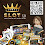## document.write(ssyby);

### Protests in Ukraine 18-20 February

The map below, based on data from here shows where the people killed in the protests of 18-20 February came from.* I can't vouch for the accuracy of the data. Apparently most of the protesters killed in Kiev come from central regions and Lviv Oblast', almost all regions are represented in the list of fatalities.

Mouse over for info about numbers and people.

The data is changing all the time - the list is twice as long as it was 24 hours ago. I will try to keep the visualization up to date.

Updated 22/02/2014

[*] People with missing 'place of origin' are not included. I removed one entry which looked like a duplicate. Age may be off by up to a year.

I transliterated names using a slightly modified Russian language transliteration scheme. I will be delighted to take corrections:

a["Ё"]="Yo";a["Й"]="I";a["Ц"]="Ts";a["У"]="U";a["К"]="K";a["Е"]="E";a["Н"]="N";a["Г"]="G";a["Ш"]="Sh";a["Щ"]="Shch";a["З"]="Z";a["Х"]="Kh";a["Ъ"]="'";a["ё"]="yo";a["й"]="i";a["ц"]="ts";a["у"]="u";a["к"]="k";a["е"]="e";a["н"]="n";a["г"]="g";a["ш"]="sh";a["щ"]="shch";a["з"]="z";a["х"]="kh";a["ъ"]="'";a["Ф"]="F";a["Ы"]="Y";a["В"]="V";a["А"]="A";a["П"]="P";a["Р"]="R";a["О"]="O";a["Л"]="L";a["Д"]="D";a["Ж"]="Zh";a["Э"]="E";a["ф"]="f";a["ы"]="y";a["в"]="v";a["а"]="a";a["п"]="p";a["р"]="r";a["о"]="o";a["л"]="l";a["д"]="d";a["ж"]="zh";a["э"]="e";a["Я"]="Ya";a["Ч"]="Ch";a["С"]="S";a["М"]="M";a["И"]="I";a["Т"]="T";a["Ь"]="'";a["Б"]="B";a["Ю"]="Yu";a["я"]="ia";a["ч"]="ch";a["с"]="s";a["м"]="m";a["и"]="i";a["т"]="t";a["ь"]="'";a["б"]="b";a["ю"]="iu";a["є"]="e";a["Є"]="Ye";a["і"]="i";a["Ї"]="Yi";

1.The ultimate article. Thank you for writing a good article to read.
สล็อตโจ๊กเกอร์

2.3.